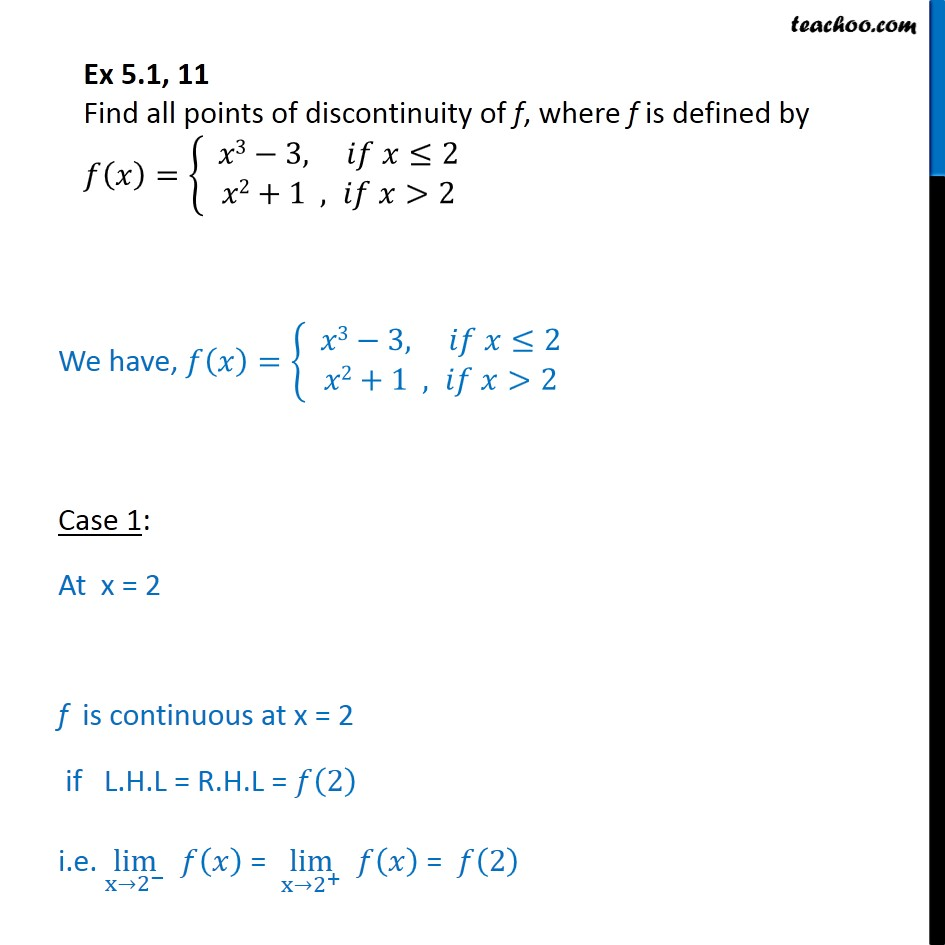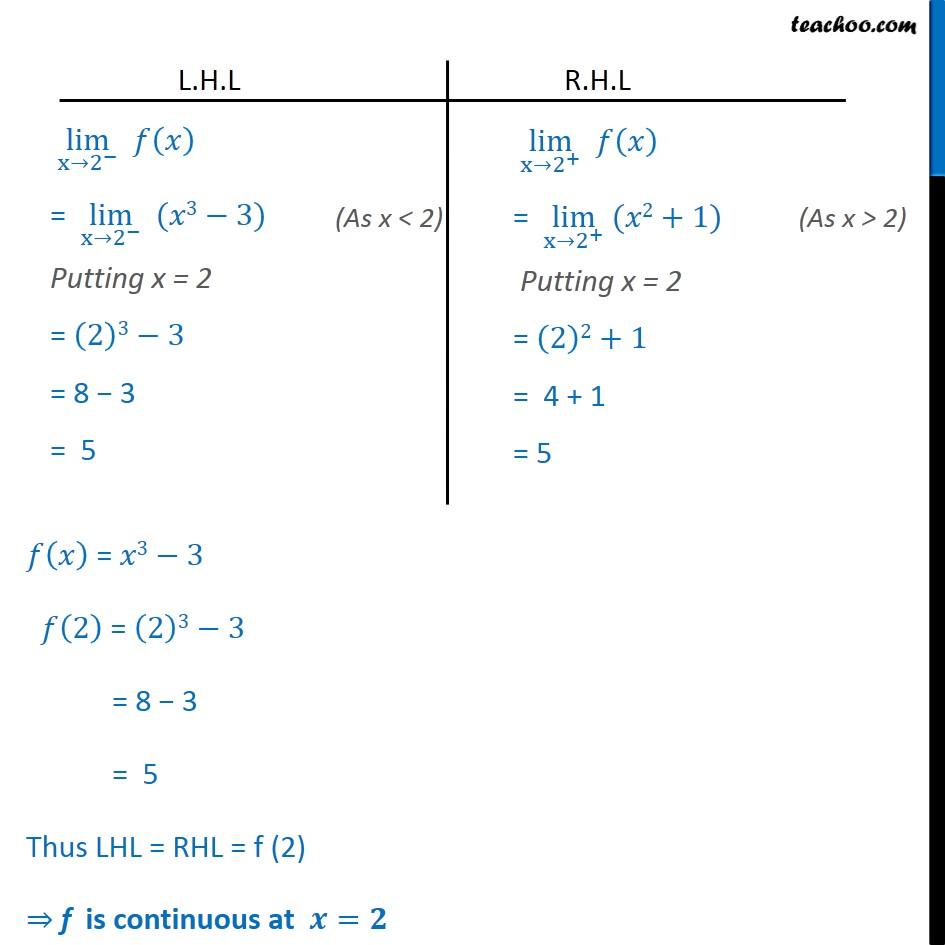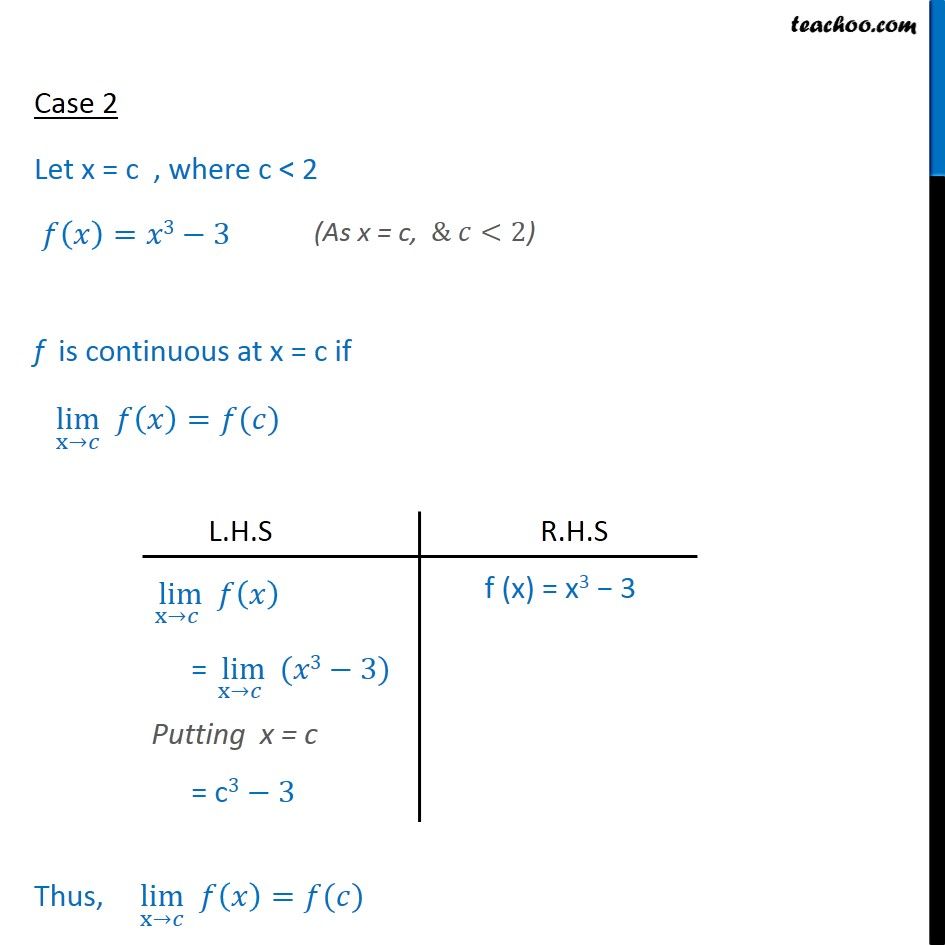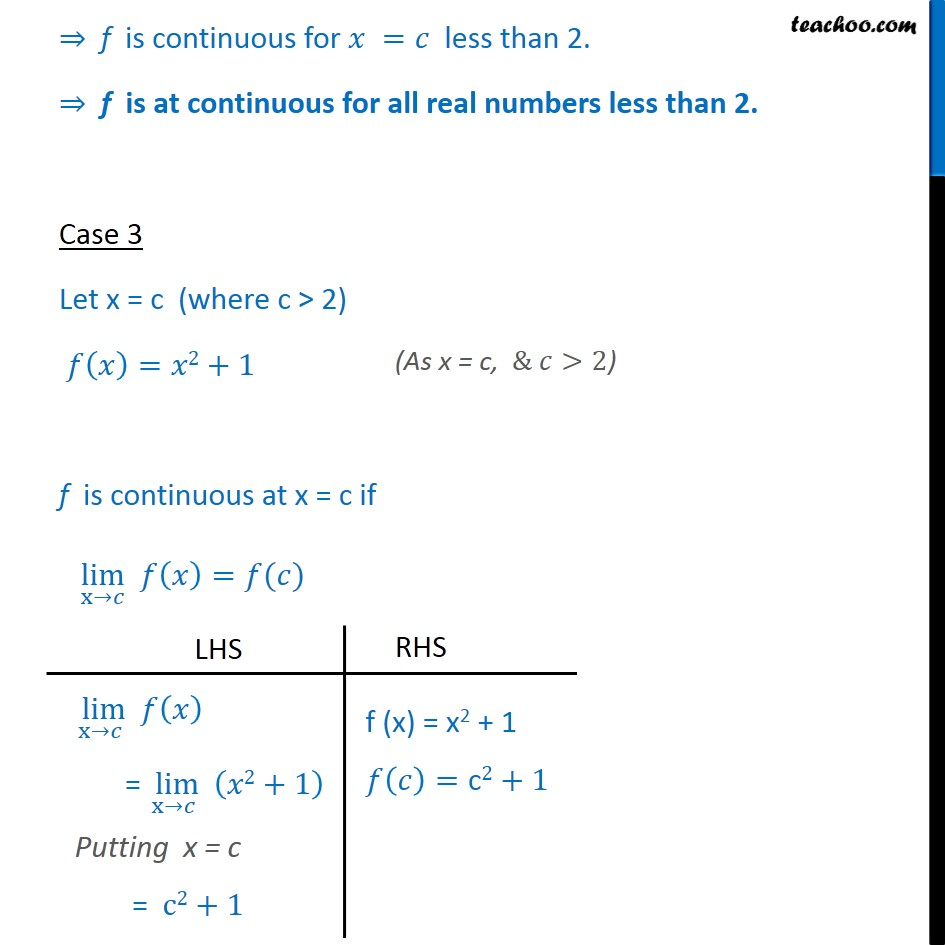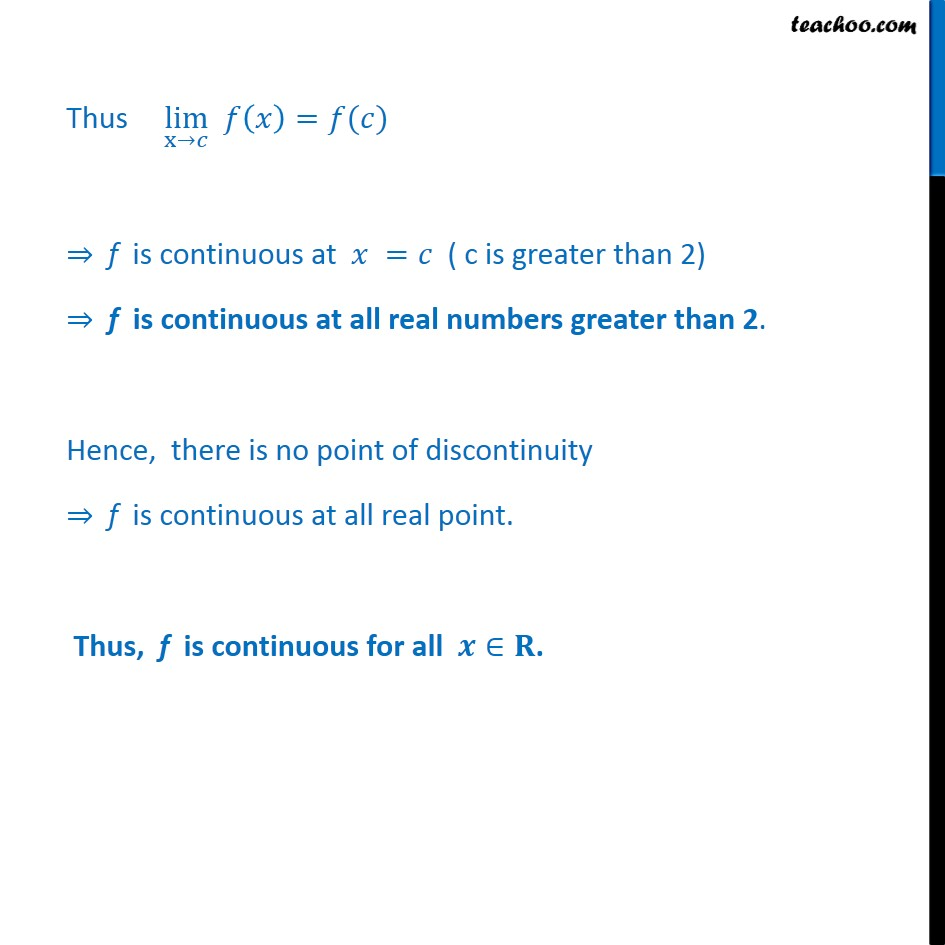1. Chapter 5 Class 12 Continuity and Differentiability
2. Serial order wise
3. Ex 5.1

Transcript

Ex 5.1, 11 Find all points of discontinuity of f, where f is defined by 𝑓 𝑥﷯= 𝑥3−3, 𝑖𝑓 𝑥≤2﷮&𝑥2+1 , 𝑖𝑓 𝑥>2﷯﷯ We have, 𝑓 𝑥﷯= 𝑥3−3, 𝑖𝑓 𝑥≤2﷮&𝑥2+1 , 𝑖𝑓 𝑥>2﷯﷯ Case 1: At x = 2 f is continuous at x = 2 if L.H.L = R.H.L = 𝑓 2﷯ i.e. lim﷮x→ 2﷮−﷯﷯ 𝑓 𝑥﷯ = lim﷮x→ 2﷮+﷯﷯ 𝑓 𝑥﷯ = 𝑓 2﷯ 𝑓 𝑥﷯ = 𝑥3−3 𝑓 2﷯ = 2﷯3−3 = 8 − 3 = 5 Thus LHL = RHL = f (2) ⇒ f is continuous at 𝒙=𝟐 Case 2 Let x = c , where c < 2 𝑓 𝑥﷯=𝑥3−3 f is continuous at x = c if lim﷮x→𝑐﷯ 𝑓 𝑥﷯=𝑓(𝑐) Thus, lim﷮x→𝑐﷯ 𝑓 𝑥﷯=𝑓(𝑐) ⇒ f is continuous for 𝑥 =𝑐 less than 2. ⇒ f is at continuous for all real numbers less than 2. Case 3 Let x = c (where c > 2) 𝑓 𝑥﷯=𝑥2+1 f is continuous at x = c if lim﷮x→𝑐﷯ 𝑓 𝑥﷯=𝑓(𝑐) Thus lim﷮x→𝑐﷯ 𝑓 𝑥﷯=𝑓(𝑐) ⇒ f is continuous at 𝑥 =𝑐 ( c is greater than 2) ⇒ f is continuous at all real numbers greater than 2. Hence, there is no point of discontinuity ⇒ f is continuous at all real point. Thus, f is continuous for all 𝒙∈𝐑.

Ex 5.1